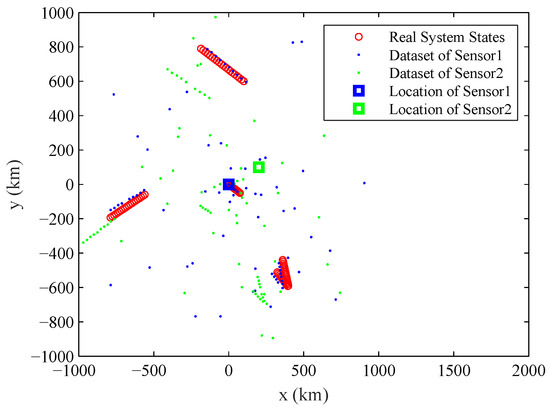Previous Article in Journal
Symbolic Entropy Analysis and Its Applications
Previous Article in Special Issue
Stochastic Proximal Gradient Algorithms for Multi-Source Quantitative Photoacoustic Tomography
Open AccessArticle

Random Finite Set Based Parameter Estimation Algorithm for Identifying Stochastic Systems

College of Systems Engineering, National University of Defense Technology, Changsha 410073, China
*
Author to whom correspondence should be addressed.
Entropy 2018, 20(8), 569; https://doi.org/10.3390/e20080569
Received: 13 June 2018 / Revised: 21 July 2018 / Accepted: 26 July 2018 / Published: 31 July 2018
(This article belongs to the Special Issue Probabilistic Methods for Inverse Problems)
Parameter estimation is one of the key technologies for system identification. The Bayesian parameter estimation algorithms are very important for identifying stochastic systems. In this paper, a random finite set based algorithm is proposed to overcome the disadvantages of the existing Bayesian parameter estimation algorithms. It can estimate the unknown parameters of the stochastic system which consists of a varying number of constituent elements by using the measurements disturbed by false detections, missed detections and noises. The models used for parameter estimation are constructed by using random finite set. Based on the proposed system model and measurement model, the key principles and formula derivation of the proposed algorithm are detailed. Then, the implementation of the algorithm is presented by using sequential Monte Carlo based Probability Hypothesis Density (PHD) filter and simulated tempering based importance sampling. Finally, the experiments of systematic errors estimation of multiple sensors are provided to prove the main advantages of the proposed algorithm. The sensitivity analysis is carried out to further study the mechanism of the algorithm. The experimental results verify the superiority of the proposed algorithm. View Full-Text
Show FiguresFigure 1

MDPI and ACS Style

Wang, P.; Li, G.; Peng, Y.; Ju, R. Random Finite Set Based Parameter Estimation Algorithm for Identifying Stochastic Systems. Entropy 2018, 20, 569.

Show more citation formats Show less citations formats
Note that from the first issue of 2016, MDPI journals use article numbers instead of page numbers. See further details here.

1